Author: Oscar Cronquist Article last updated on April 25, 2019

Hi Oscar,
I need a fomula that gives me the number of days contained in a range that overlap another range... not sure if that is clear enough...

I got this from Carol Weideman, thank you! I think it is really simple and clever.

Date range 1: A to B

Date range 2: C to D

Formula: =MEDIAN(A,B+1,D+1)-MEDIAN(A,B+1,C)

MEDIAN(number1, [number2], ..)
Returns the median, or the number in the middle of the set of given numbers

### Example 1 - No overlapping date ranges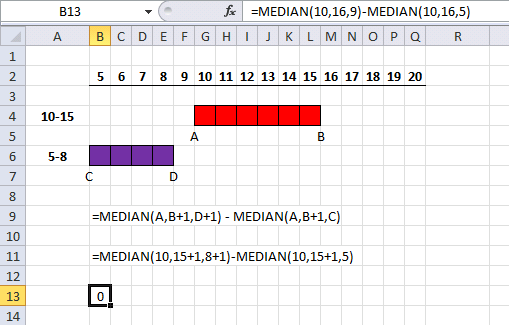=MEDIAN(A,B+1,D+1) - MEDIAN(A,B+1,C)

becomes

=MEDIAN(10,15+1,8+1)-MEDIAN(10,15+1,5)

becomes

=MEDIAN(10,16,9)-MEDIAN(10,16,5)

becomes

10 - 10 = 0

0 days are overlapping.

### Example 2 - Partially overlapping date ranges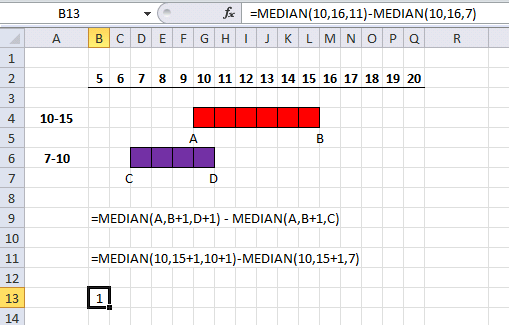=MEDIAN(A,B+1,D+1) - MEDIAN(A,B+1,C)

becomes

=MEDIAN(10,15+1,10+1)-MEDIAN(10,15+1,7)

becomes

=MEDIAN(10,16,11)-MEDIAN(10,16,7)

becomes

11 - 10 = 1

1 day is overlapping

### Example 3 - Overlapping date ranges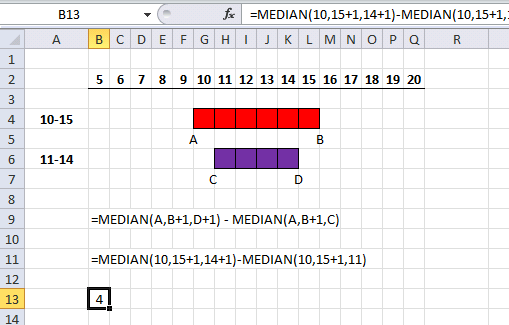=MEDIAN(A,B+1,D+1) - MEDIAN(A,B+1,C)

becomes

=MEDIAN(10,15+1,14+1)-MEDIAN(10,15+1,11)

becomes

=MEDIAN(10,16,15)-MEDIAN(10,16,11)

becomes

15 - 11 = 4

4 days are overlapping

### Example 4 - Partially overlapping date ranges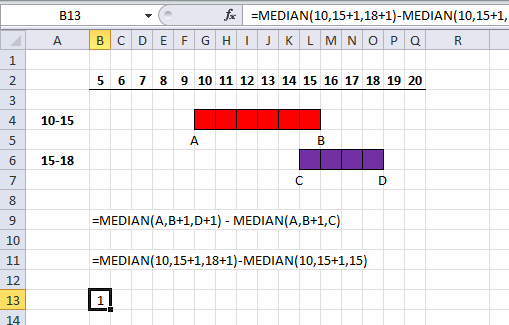=MEDIAN(A,B+1,D+1) - MEDIAN(A,B+1,C)

becomes

=MEDIAN(10,15+1,18+1)-MEDIAN(10,15+1,15)

becomes

=MEDIAN(10,16,19)-MEDIAN(10,16,15)

becomes

16 - 15 = 1

1 day is overlapping

### Example 5 - No overlapping date ranges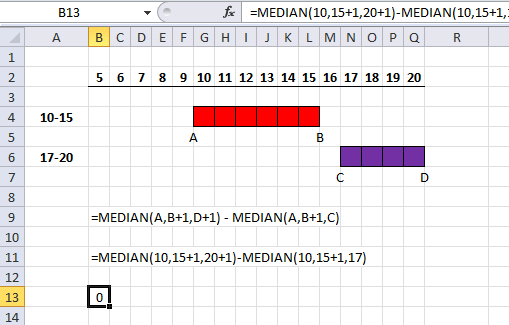=MEDIAN(A,B+1,D+1) - MEDIAN(A,B+1,C)

becomes

=MEDIAN(10,15+1,20+1)-MEDIAN(10,15+1,17)

becomes

=MEDIAN(10,16,21)-MEDIAN(10,16,17)

becomes

16 - 16 = 0

0 days are overlapping